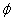NEET  >  Test: Motional Emf

# Test: Motional Emf

Test Description

## 10 Questions MCQ Test Physics Class 12 | Test: Motional Emf

Test: Motional Emf for NEET 2023 is part of Physics Class 12 preparation. The Test: Motional Emf questions and answers have been prepared according to the NEET exam syllabus.The Test: Motional Emf MCQs are made for NEET 2023 Exam. Find important definitions, questions, notes, meanings, examples, exercises, MCQs and online tests for Test: Motional Emf below.
Solutions of Test: Motional Emf questions in English are available as part of our Physics Class 12 for NEET & Test: Motional Emf solutions in Hindi for Physics Class 12 course. Download more important topics, notes, lectures and mock test series for NEET Exam by signing up for free. Attempt Test: Motional Emf | 10 questions in 10 minutes | Mock test for NEET preparation | Free important questions MCQ to study Physics Class 12 for NEET Exam | Download free PDF with solutions
 1 Crore+ students have signed up on EduRev. Have you?
Test: Motional Emf - Question 1

### The magnetic flux passing normally through a coil is ø = 20 cos πt + t2 + 50t + 25m Wb What will be the induced emf at t = 1s?​

Detailed Solution for Test: Motional Emf - Question 1

θ=20cos5πt+t2+50t+25 mWb
We know that,
Emf=dθ/dt=-20x5πsin5πt+2t+50+0
At,t=1
Emf=-100πsing5π+2+50
=0+2+50
=52V

Test: Motional Emf - Question 2

### A copper rod moves parallel to the horizontal direction. The induced emf developed across its ends due earth’s magnetic field will be maximum at the

Detailed Solution for Test: Motional Emf - Question 2

At the poles of the copper rod, the earth’s magnetic field will be more. This is explained with the help of Lorentz force where he explains that the magnetic field is generated due to the movement of charges.

Earth’s magnetic field is defined as the magnetic dipole which is similar to bar magnet and is tilted 11° from the earth’s axis of rotation.

Test: Motional Emf - Question 3

### The magnetic flux through a surface varies with time as follows:= 12t2 + 7t + 3 here,is in milliweber and t is in seconds. What will be the induced emf at t = 5s?​

Detailed Solution for Test: Motional Emf - Question 3

Given :
ϕ=12t2+7t+C
where, C is a constant, t is in sec, ϕ in milli-weber
The induced e.m.f. in the loop at t=5, on differentiating the equation with respect to 't'.
dtdϕ​=dtd​(12t2+7t+C)
=24t+7
at            t=5
dtdϕ​=24×(5)+7
=120+7
=127 mV

Test: Motional Emf - Question 4

In Faraday’s experiment if the magnet is moved towards the coil, it results in ____________ in magnetic field B at any point on the wire loop. The _________ shows deflection. Thus emf is induced by changing B.

Test: Motional Emf - Question 5

What is the value of induced e.m.f if a wire is moved between the gap of two poles of a magnetin in 1 s and magnetic flux between the poles is 6 x 10-3 Wb?​

Test: Motional Emf - Question 6

Two similar circular co-axial loops carry equal current in the same direction. If the loops be brought nearer, the currents in them will

Detailed Solution for Test: Motional Emf - Question 6

When the two loops are brought together, the flux linking the two loops increases. By Lenz's law a current will be induced such that it opposes this change of flux . Therefore to oppose the increase in flux, the current in the two loops will decrease, so the flux decreases.

Test: Motional Emf - Question 7

When a wire loop is rotated in a magnetic field, the direction of induced emf changes once in each

Detailed Solution for Test: Motional Emf - Question 7

Flux of the magnetic field through the loop
ϕ=B.Acosωt
Emf= −dϕ/dt​=B.Aωsinωt
ωt=0 to π,  sinωt=+ve
ωt=π to2 π,  sinωt=−ve
Hence, the direction of induced e.m.f. changes once in 1/2​ revolution.

Test: Motional Emf - Question 8

The induced e.m.f in a coil is given by ε = 100sin 100π volts. Calculate the number of rotations the coil makes per second.​

Detailed Solution for Test: Motional Emf - Question 8

ε=100sinπV
ε=ε0sinωt
ω=100π
2πn=100π
n=50rps

Test: Motional Emf - Question 9

The motional emf produced across a wire moving through a magnetic field does not depend on its:

Detailed Solution for Test: Motional Emf - Question 9

Motional emf= Bℓv
It depends on magnetic field intensity, length and velocity. The diameter doesn't affect any of the factors.

Test: Motional Emf - Question 10

If we keep two similar loops made up of copper and constantan in a magnetic field for same amount of time, in which loop the induced e.m.f will be greater.

Detailed Solution for Test: Motional Emf - Question 10

As the copper has high electrical conductivity. Hence, higher e.m.f would be induced in the copper loop.

## Physics Class 12

157 videos|452 docs|213 tests
 Use Code STAYHOME200 and get INR 200 additional OFF Use Coupon Code
Information about Test: Motional Emf Page
In this test you can find the Exam questions for Test: Motional Emf solved & explained in the simplest way possible. Besides giving Questions and answers for Test: Motional Emf, EduRev gives you an ample number of Online tests for practice

## Physics Class 12

157 videos|452 docs|213 tests

### How to Prepare for NEET

Read our guide to prepare for NEET which is created by Toppers & the best Teachers